# EDA, FE, HPO - All you need (LB: 0.640)

Detailed EDA, FE with Class Balancing, Hyper-Parameter Optimization of XGBoost using Optuna

This notebook explains feature-level exploratory data analysis along with observation comments, simple feature engineering including class balancing and XGBoost hyper-parameter optimization using HPO framework Optuna.# What is the notebook about?¶

The challenge is to use the features extracted from the Clock Drawing Test to build an automated and algorithm to predict whether each participant is one of three phases:

1) Pre-Alzheimer’s (Early Warning) 2) Post-Alzheimer’s (Detection) 3) Normal (Not an Alzheimer’s patient)

In machine learning terms: this is a 3-class classification task.

# How to use this notebook? 📝¶

• Update the config parameters. You can define the common variables here
Variable Description
`AICROWD_DATASET_PATH` Path to the file containing test data (The data will be available at `/ds_shared_drive/` on aridhia workspace). This should be an absolute path.
`AICROWD_PREDICTIONS_PATH` Path to write the output to.
`AICROWD_ASSETS_DIR` In case your notebook needs additional files (like model weights, etc.,), you can add them to a directory and specify the path to the directory here (please specify relative path). The contents of this directory will be sent to AIcrowd for evaluation.
`AICROWD_API_KEY` In order to submit your code to AIcrowd, you need to provide your account's API key. This key is available at https://www.aicrowd.com/participants/me
• Installing packages. Please use the Install packages 🗃 section to install the packages
• Training your models. All the code within the Training phase ⚙️ section will be skipped during evaluation. Please make sure to save your model weights in the assets directory and load them in the predictions phase section

# Content:¶

• Exploratory Data Analysis
• Feature Engineering
• Hyper-parameter Optimization
• Training Best Parameters Model
• Final Prediction and submission

### Introduction:¶

Hello I am Jyot Makadiya, a pre-final year student pursuing bachelor of technology in computer science & engineering. I have been experimenting with data for 1 year now and so far the journey has been smooth and I learned a lot on the way.
This challenge can be assumed to be a multiclass classification problem with 3 classes ( Normal, Pre-Alzheimer’s, Post-Alzheimer’s). The main tasks to achieve a good score include having a good cross-validation with balanced dataset, good feature engineering and Fine-tuning hyper-parameters along with ensembling. </br>
This notebook covers my approach for this competition starting with exploratory data analysis. Then it covers simple feature engineering for a few features (I'll expand the idea of FE and ensemble in next part/walkthrough blog). Finally we use Optuna for hyper-parameter optimization. </br>
The aim of this notebook is to introduce you with the variety of concepts including but not limited to hyper-parameter optimization aka AutoML tools, Simple but feature level EDA and FE.
</br> For a better view of graphs and plots, open this notebook in colab using `open in colab` button

# Setup AIcrowd Utilities 🛠¶

We use this to bundle the files for submission and create a submission on AIcrowd. Do not edit this block.

In [ ]:
```!pip install -q -U aicrowd-cli
```
In [ ]:
```%load_ext aicrowd.magic
```

# AIcrowd Runtime Configuration 🧷¶

Define configuration parameters. Please include any files needed for the notebook to run under `ASSETS_DIR`. We will copy the contents of this directory to your final submission file 🙂

The dataset is available under `/ds_shared_drive` on the workspace.

In [ ]:
```import os

# Please use the absolute for the location of the dataset.
# Or you can use relative path with `os.getcwd() + "test_data/validation.csv"`
AICROWD_DATASET_PATH = os.getenv("DATASET_PATH", "Z:/challenge-data/validation.csv")
AICROWD_PREDICTIONS_PATH = os.getenv("PREDICTIONS_PATH", "Z:/challenge-data/predictions.csv")
AICROWD_ASSETS_DIR = "assets"
```

# Install packages 🗃¶

In [ ]:
```!pip install -q numpy pandas
```
In [ ]:
```!pip install -q xgboost scikit-learn seaborn lightgbm optuna
```

# Define preprocessing code 💻¶

The code that is common between the training and the prediction sections should be defined here. During evaluation, we completely skip the training section. Please make sure to add any common logic between the training and prediction sections here.

### Import common packages¶

Please import packages that are common for training and prediction phases here.

In [ ]:
```import xgboost as xgb
import numpy as np
import pandas as pd
import pickle
import seaborn as sns
import matplotlib.pyplot as plt
%matplotlib inline
sns.set()
sns.color_palette("rocket_r")
pd.set_option('display.max_rows', 200)
pd.set_option('display.max_columns', 200)
pd.set_option('display.width', 1000)

import lightgbm as lgb
from sklearn.model_selection import train_test_split
from sklearn.model_selection import StratifiedKFold
from sklearn.metrics import accuracy_score, log_loss, f1_score
import joblib

import warnings
warnings.filterwarnings("ignore")
# df
# with open(AICROWD_DATASET_PATH) as f:
# some precessing code
```
In [ ]:
```# os.listdir('Z:/challenge-data/')
#Pre Processing functions
```

# Training phase ⚙️¶

You can define your training code here. This sections will be skipped during evaluation.

In [ ]:
```# model = define_your_model
```

In [ ]:
```df_orig = pd.read_csv("Z:/challenge-data/train.csv")

df = df_orig.copy()
df.describe()
```
Out[ ]:
number_of_digits missing_digit_1 missing_digit_2 missing_digit_3 missing_digit_4 missing_digit_5 missing_digit_6 missing_digit_7 missing_digit_8 missing_digit_9 missing_digit_10 missing_digit_11 missing_digit_12 1 dist from cen 10 dist from cen 11 dist from cen 12 dist from cen 2 dist from cen 3 dist from cen 4 dist from cen 5 dist from cen 6 dist from cen 7 dist from cen 8 dist from cen 9 dist from cen euc_dist_digit_1 euc_dist_digit_2 euc_dist_digit_3 euc_dist_digit_4 euc_dist_digit_5 euc_dist_digit_6 euc_dist_digit_7 euc_dist_digit_8 euc_dist_digit_9 euc_dist_digit_10 euc_dist_digit_11 euc_dist_digit_12 area_digit_1 area_digit_2 area_digit_3 area_digit_4 area_digit_5 area_digit_6 area_digit_7 area_digit_8 area_digit_9 area_digit_10 area_digit_11 area_digit_12 height_digit_1 height_digit_2 height_digit_3 height_digit_4 height_digit_5 height_digit_6 height_digit_7 height_digit_8 height_digit_9 height_digit_10 height_digit_11 height_digit_12 width_digit_1 width_digit_2 width_digit_3 width_digit_4 width_digit_5 width_digit_6 width_digit_7 width_digit_8 width_digit_9 width_digit_10 width_digit_11 width_digit_12 variance_width variance_height variance_area deviation_dist_from_mid_axis between_axis_digits_angle_sum between_axis_digits_angle_var between_digits_angle_cw_sum between_digits_angle_cw_var between_digits_angle_ccw_sum between_digits_angle_ccw_var sequence_flag_cw sequence_flag_ccw number_of_hands hand_count_dummy hour_hand_length minute_hand_length single_hand_length clockhand_ratio clockhand_diff angle_between_hands deviation_from_centre hour_proximity_from_11 minute_proximity_from_2 hour_pointing_digit actual_hour_digit minute_pointing_digit actual_minute_digit final_rotation_angle ellipse_circle_ratio count_defects percentage_inside_ellipse pred_tremor double_major double_minor vertical_dist horizontal_dist top_area_perc bottom_area_perc left_area_perc right_area_perc hor_count vert_count eleven_ten_error other_error time_diff centre_dot_detect
count 32703.000000 32703.000000 32703.000000 32703.000000 32703.000000 32703.000000 32703.000000 32703.000000 32703.000000 32703.000000 32703.000000 32703.000000 32703.000000 25448.000000 27882.000000 27201.000000 28937.000000 27855.000000 28612.000000 27251.000000 26092.000000 28407.000000 28555.000000 28755.000000 26974.000000 25400.000000 27800.000000 28603.000000 27238.000000 26082.000000 28394.000000 28491.000000 28641.000000 2.693600e+04 27838.000000 27151.000000 28909.000000 25448.000000 27855.000000 28612.000000 27251.000000 26092.000000 28407.000000 28555.000000 28755.000000 26974.000000 27882.000000 27201.000000 28937.000000 25448.000000 27855.000000 28612.000000 27251.000000 26092.000000 28407.000000 28555.000000 28755.000000 26974.000000 27882.000000 27201.000000 28937.000000 25448.000000 27855.000000 28612.000000 27251.000000 26092.000000 28407.000000 28555.000000 28755.000000 26974.000000 27882.000000 27201.000000 28937.000000 32313.000000 32313.000000 3.231300e+04 32198.000000 29141.000000 30870.000000 20027.000000 32085.000000 844.000000 32085.000000 32474.000000 32474.000000 30623.000000 30623.000000 22861.000000 22861.000000 7741.000000 22650.000000 22835.000000 22861.000000 22793.000000 20191.000000 19919.000000 22677.000000 32777.0 22678.000000 32777.0 32703.000000 3.203900e+04 32777.000000 32472.000000 32777.000000 32540.000000 3.277600e+04 3.258900e+04 3.273500e+04 31218.000000 31218.000000 31218.000000 31218.000000 32777.000000 32777.000000 32777.000000 32777.000000 22526.000000 22826.000000
mean 10.299422 0.221845 0.148243 0.125096 0.166713 0.202153 0.131364 0.126839 0.120723 0.175183 0.147418 0.168241 0.115158 361.869732 367.418424 368.235873 370.796838 349.116177 337.542587 336.085919 335.550313 353.017822 368.547709 370.329200 375.631690 30.287315 32.834984 33.031035 32.049520 30.724226 28.135344 30.886070 32.250843 3.125026e+01 33.247571 32.644335 28.629239 2308.107671 4616.101562 5046.115231 5793.115665 7214.179250 6035.063259 4942.821748 5697.203373 5678.539964 6647.253927 5393.460167 6998.064450 59.880541 70.994184 80.247973 87.709479 88.637130 88.011054 81.457468 85.024761 89.002818 81.400330 75.654792 77.071742 40.342109 62.648717 61.032259 65.411545 79.223402 68.243391 60.447242 66.558233 63.786943 78.795890 69.117569 87.386426 363.578878 324.115546 5.148403e+06 32.202820 352.139508 2587.128279 355.100767 3081.777480 243.825427 3157.421099 0.750385 0.018538 1.772132 1.770369 60.538409 80.874117 74.602333 1.375478 20.270851 90.170001 17.420096 24.922338 33.267558 9.047449 11.0 4.393377 2.0 65.737088 7.911654e+01 93.489459 0.939555 0.317052 120.238950 1.065362e+02 1.115766e+02 1.139164e+02 0.519007 0.465878 0.525769 0.464433 0.693230 0.762211 0.025231 0.612655 105.199325 0.241172
std 2.345710 0.415494 0.355346 0.330832 0.372725 0.401612 0.337803 0.332797 0.325810 0.380129 0.354527 0.374086 0.319217 50.310698 48.060878 48.425983 48.005863 53.313076 51.175381 47.456872 46.910977 47.096105 50.956366 51.562665 45.795291 33.877417 31.828580 33.060628 31.662544 30.055328 31.245333 33.028061 33.840305 3.440961e+01 34.375507 34.165306 35.018626 1070.213451 2365.657591 2569.549735 2641.521129 3474.474015 2742.576668 2221.963276 2741.527329 2563.651971 3161.975614 2633.392241 3525.529979 20.742269 21.127381 23.071334 26.389729 23.653629 27.994521 24.236242 25.677814 27.629884 21.714878 20.775003 21.834246 17.562823 17.698763 19.128573 19.661431 24.229453 21.233585 19.571260 22.255969 21.164594 21.110245 20.374876 25.649241 306.449113 302.509846 6.805541e+06 32.276635 52.263430 5675.602203 41.688130 5648.419057 168.298221 5784.787525 0.432797 0.134888 0.457020 0.448046 14.191507 13.311371 35.873579 0.299056 13.359890 23.522379 19.001146 39.291724 46.534051 3.661448 0.0 3.965286 0.0 110.472325 1.453976e+01 39.504488 0.169569 0.465335 19.864539 1.295960e+01 1.900768e+01 1.467719e+01 0.180912 0.178807 0.160916 0.160926 0.675787 0.699355 0.156829 0.487151 205.429390 0.427804
min 1.000000 0.000000 0.000000 0.000000 0.000000 0.000000 0.000000 0.000000 0.000000 0.000000 0.000000 0.000000 0.000000 3.354102 5.852350 11.335784 22.102036 7.905694 15.206906 6.519202 7.826238 3.535534 14.422205 8.139410 14.115594 0.000760 0.003261 0.000000 0.000760 0.002345 0.000000 0.000491 0.000515 2.960000e-14 0.002010 0.001071 0.000000 640.000000 768.000000 828.000000 1036.000000 1152.000000 805.000000 777.000000 1054.000000 870.000000 888.000000 780.000000 1089.000000 19.000000 21.000000 29.000000 31.000000 30.000000 28.000000 24.000000 28.000000 24.000000 31.000000 26.000000 28.000000 18.000000 26.000000 23.000000 26.000000 32.000000 23.000000 21.000000 24.000000 25.000000 24.000000 24.000000 30.000000 0.000000 0.000000 0.000000e+00 0.000000 0.000000 0.000000 0.000000 0.000000 0.000000 0.000000 0.000000 0.000000 1.000000 1.000000 23.164818 33.186431 18.017081 1.000000 0.000000 0.029409 0.100852 0.000000 0.000000 1.000000 11.0 1.000000 2.0 0.000000 5.060000e-10 1.000000 0.000000 0.000000 9.696612 4.210000e-10 4.210000e-10 2.600000e-09 0.000000 0.000000 0.000000 0.000000 0.000000 0.000000 0.000000 0.000000 -110.000000 0.000000
25% 10.000000 0.000000 0.000000 0.000000 0.000000 0.000000 0.000000 0.000000 0.000000 0.000000 0.000000 0.000000 0.000000 336.580321 343.945581 342.212288 348.353987 320.153479 308.950947 309.714788 309.358914 328.613374 342.429190 345.253711 353.542784 6.680591 8.930655 8.190000 8.141702 7.908380 6.240000 6.903923 7.413237 6.890000e+00 7.541077 7.369695 5.200000 1537.000000 2942.000000 3240.000000 3915.000000 4758.000000 4041.000000 3312.000000 3760.000000 3848.000000 4380.000000 3540.000000 4473.000000 45.000000 56.000000 63.000000 69.000000 72.000000 67.000000 63.000000 67.000000 69.000000 66.000000 61.000000 62.000000 29.000000 50.000000 47.000000 52.000000 61.000000 54.000000 47.000000 50.000000 50.000000 64.000000 54.000000 69.000000 171.515152 148.277778 1.575504e+06 9.880000 360.000000 102.884207 360.000000 51.690940 0.000000 52.068825 1.000000 0.000000 2.000000 2.000000 50.270850 71.700628 53.825024 1.138048 9.375855 82.023132 8.079066 2.334051 1.728408 10.000000 11.0 2.000000 2.0 0.000000 7.802811e+01 78.000000 1.000000 0.000000 115.425250 1.027451e+02 1.077028e+02 1.099097e+02 0.472774 0.480002 0.502471 0.457726 0.000000 0.000000 0.000000 0.000000 0.000000 0.000000
50% 11.000000 0.000000 0.000000 0.000000 0.000000 0.000000 0.000000 0.000000 0.000000 0.000000 0.000000 0.000000 0.000000 367.434688 372.683512 372.667412 377.180328 353.802911 343.432854 340.306479 339.694716 358.558224 372.874309 375.153635 381.106284 14.935799 20.012485 18.980000 19.097071 18.527444 14.300000 15.724508 16.984068 1.586000e+01 17.561152 17.209259 11.960000 2065.000000 4104.000000 4508.000000 5270.000000 6525.000000 5580.000000 4559.000000 5145.000000 5280.000000 6075.000000 4872.000000 6240.000000 59.000000 68.000000 78.000000 86.000000 87.000000 86.000000 80.000000 84.000000 88.000000 79.000000 73.000000 74.000000 34.000000 60.000000 57.000000 61.000000 75.000000 64.000000 57.000000 62.000000 59.000000 76.000000 66.000000 84.000000 282.787879 246.386364 3.114518e+06 16.163333 360.000000 296.051705 360.000000 165.733916 360.000000 167.238094 1.000000 0.000000 2.000000 2.000000 59.852542 80.769660 68.324616 1.307708 18.837195 91.649346 13.104333 5.189904 4.258455 11.000000 11.0 2.000000 2.0 0.000000 8.375303e+01 103.000000 1.000000 0.000000 120.289760 1.093602e+02 1.130389e+02 1.160801e+02 0.493087 0.505231 0.520833 0.478135 1.000000 1.000000 0.000000 1.000000 0.000000 0.000000
75% 12.000000 0.000000 0.000000 0.000000 0.000000 0.000000 0.000000 0.000000 0.000000 0.000000 0.000000 0.000000 0.000000 393.898464 397.112940 399.011278 401.186366 383.428285 371.767737 367.008855 366.316888 384.157520 400.218222 401.983364 404.633476 37.094044 46.669563 47.840000 45.860710 44.763078 35.100000 44.079657 46.748061 4.085250e+01 50.597531 48.329409 31.330000 2816.000000 5716.000000 6231.000000 7105.500000 8840.000000 7560.000000 6120.000000 7000.000000 7038.000000 8232.000000 6640.000000 8701.000000 73.000000 83.000000 94.000000 104.000000 103.000000 107.000000 98.000000 101.000000 107.000000 94.000000 88.000000 89.000000 47.000000 72.000000 71.000000 75.000000 93.000000 78.000000 70.000000 78.000000 72.000000 91.000000 80.000000 102.000000 456.363636 404.100000 6.090066e+06 44.200000 360.000000 2402.861831 360.000000 5215.235174 360.000000 5224.038548 1.000000 0.000000 2.000000 2.000000 70.048169 89.989302 85.473987 1.542887 29.315735 101.621967 20.451729 16.677090 77.721980 11.000000 11.0 10.000000 2.0 90.000000 8.742738e+01 121.000000 1.000000 1.000000 124.313176 1.135460e+02 1.163918e+02 1.201251e+02 0.516964 0.525196 0.540867 0.496303 1.000000 1.000000 0.000000 1.000000 60.000000 0.000000
max 17.000000 1.000000 1.000000 1.000000 1.000000 1.000000 1.000000 1.000000 1.000000 1.000000 1.000000 1.000000 1.000000 618.025889 628.776988 613.843832 659.571073 568.624876 611.333379 580.975473 520.454849 586.950168 666.132119 608.481717 620.016935 119.957644 119.906309 119.860000 119.937391 119.643227 119.730000 119.997479 119.838808 1.199900e+02 119.915642 119.855309 119.990000 9870.000000 25088.000000 31482.000000 29946.000000 32200.000000 27378.000000 22866.000000 29503.000000 34524.000000 35280.000000 30338.000000 28362.000000 143.000000 213.000000 212.000000 241.000000 218.000000 225.000000 195.000000 256.000000 271.000000 213.000000 241.000000 287.000000 164.000000 193.000000 185.000000 204.000000 220.000000 209.000000 206.000000 277.000000 207.000000 219.000000 197.000000 220.000000 5408.000000 6844.500000 1.530196e+08 125.710000 360.000000 63116.006320 360.000000 63259.726600 360.000000 63259.726600 1.000000 1.000000 8.000000 3.000000 123.519704 133.691585 292.853059 2.498143 69.872251 179.518624 298.723197 179.774464 179.928116 12.000000 11.0 12.000000 2.0 330.000000 9.997281e+01 176.000000 1.000000 1.000000 499.391604 3.051857e+02 4.993892e+02 4.928847e+02 1.000000 1.000000 1.000000 1.000000 3.000000 3.000000 1.000000 1.000000 605.000000 1.000000
In [ ]:
```# list(df.columns)
```

# Exploratory Data Analysis¶

In [ ]:
```# Final Rotation Angle in degrees

feat_col = df['final_rotation_angle']
feat_col.fillna(-5,inplace=True)
plt.figure(figsize=(14,8))
fig = sns.countplot(x = 'final_rotation_angle',data=df, palette='rocket_r', hue='diagnosis')
fig.set_xlabel("Rotation Angle in Degree",size=15)
fig.set_ylabel("Angle Frequency",size=15)
plt.title('Angle frequencies for all samples',size = 20)
plt.show()
```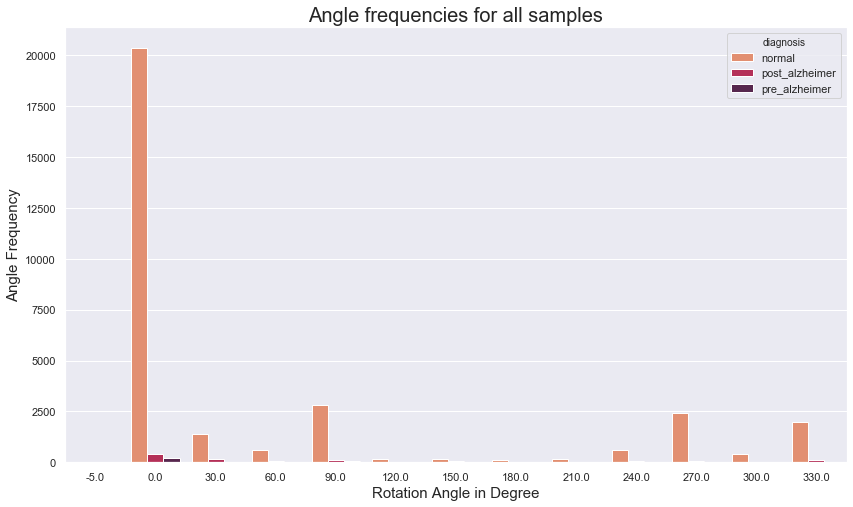We can notice that there are only 13 discrete values in rotation angles, instead of using these, we can resample that to 4 different columns each representing 90 degrees range or 1 quarter of circle angles.

In [ ]:
```print(f"number of unique values for rotation angles: {feat_col.nunique()}")

#now we can change that to 4 different quarters columns
df['rotation_angle_90'] = (feat_col <= 90).astype('int')    #we will also include NaN in this column
df['rotation_angle_180'] = (90 < feat_col) & (feat_col <= 180).astype('int')
df['rotation_angle_270'] = (180 < feat_col) & (feat_col <= 270).astype('int')
df['rotation_angle_360'] = (feat_col > 270).astype('int')

#We care not using this currently instead we will use two columns for below 180 and above 180
```
```number of unique values for rotation angles: 13
```
In [ ]:
```# number of digits
feat_col = df['number_of_digits']
feat_col.fillna(-1,inplace=True)
plt.figure(figsize=(14,8))
fig = sns.countplot(data=df, x="number_of_digits",palette='rocket', hue="diagnosis" )
fig.set_xlabel("number of digits",size=15)
fig.set_ylabel("Digits Frequency",size=15)
plt.title('Num Digits frequencies for all samples',size = 20)
plt.show()
```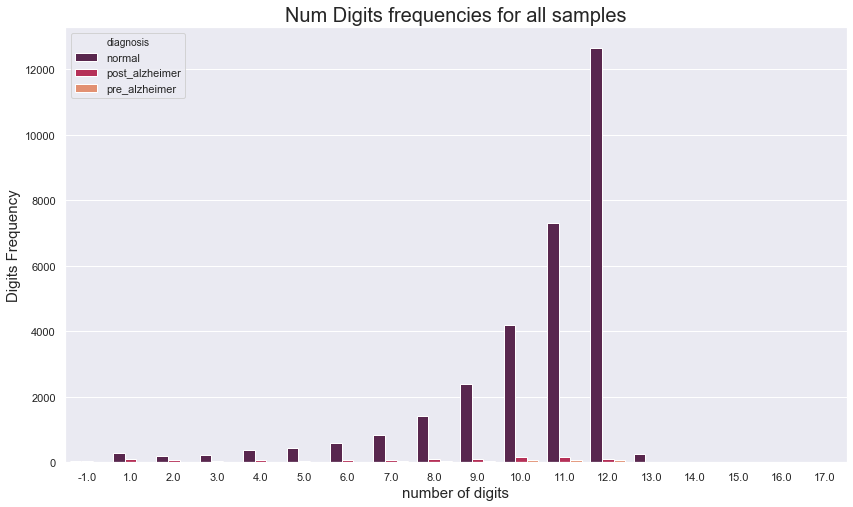In [ ]:
```print(f"number of unique values for number digits: {df['number_of_digits'].nunique()}")
```
```number of unique values for number digits: 18
```

We can notice that most of the values lie in 10,11,12 count range which is good indicator for large normal part of our dataset. And so maybe a new feature with either 10 or 11 or 12 true maybe useful

In [ ]:
```#Let's look at some of the features with categorical values of repeating multiple instances
#For missing Digit values
plt.figure()
fig, ax = plt.subplots(4, 3,figsize=(14, 20))
cont_features = [f"missing_digit_{i}" for i in range(1,13)]
for i,feature in enumerate(cont_features):
plt.subplot(4, 3,i+1)
sns.countplot(data=df, x=feature,palette='rocket' )
plt.xlabel(f"Count of values for {feature}", fontsize=12);# plt.legend()
plt.show()
```
`<Figure size 432x288 with 0 Axes>`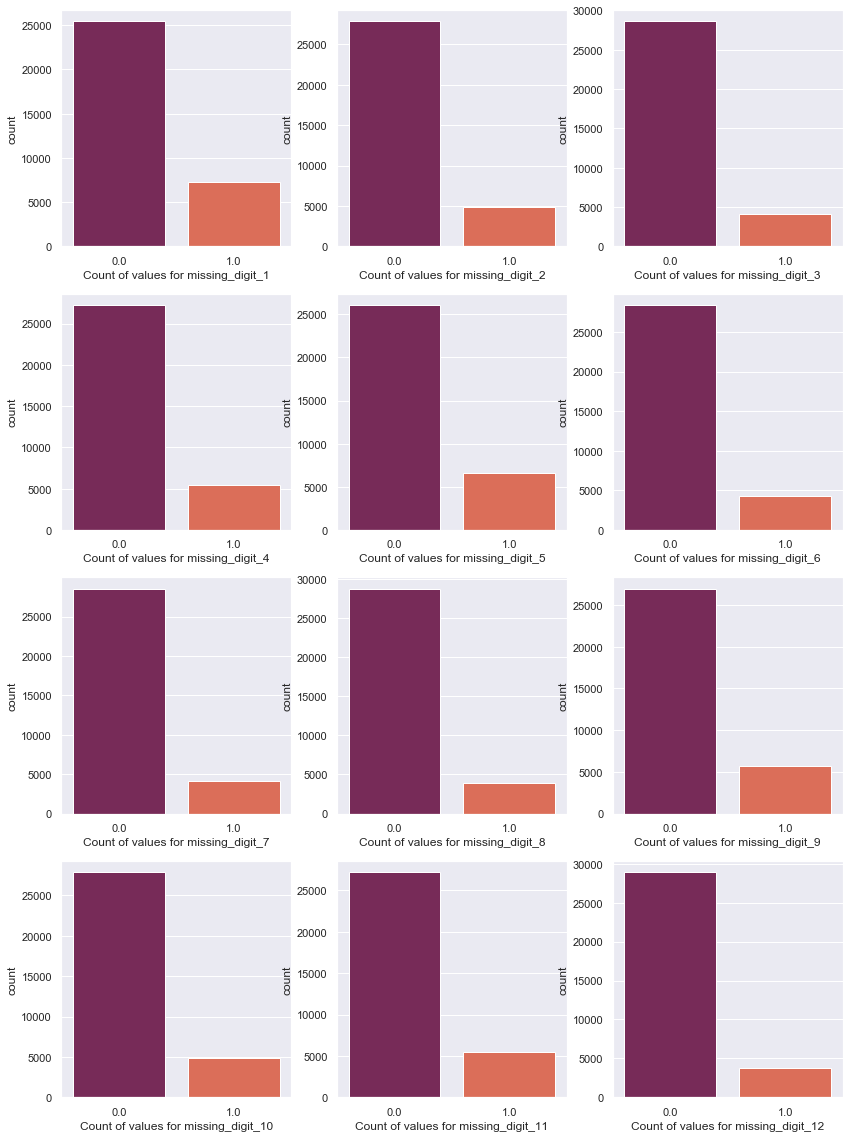The ratio is same for almost all the digits with around 5000 values being missing. We can notice the large portion in missing_digit_1 & missing_digit_5 variable

In [ ]:
```#Let's look at Euclidean distance from digits
#this feature can be calculated using Euclidean distance forumula for ideal and found digit positions with sqrt(a^2+b^2)

plt.figure()
fig, ax = plt.subplots(4, 3,figsize=(14, 20))
cont_features = [f"euc_dist_digit_{i}" for i in range(1,13)]
for i,feature in enumerate(cont_features):
plt.subplot(4, 3,i+1)
df[feature].fillna(-10,inplace=True)
sns.distplot(df[feature] , color='Red')
plt.xlabel(f"Frequency of values for {feature}", fontsize=12);# plt.legend()
plt.show()
```
`<Figure size 432x288 with 0 Axes>`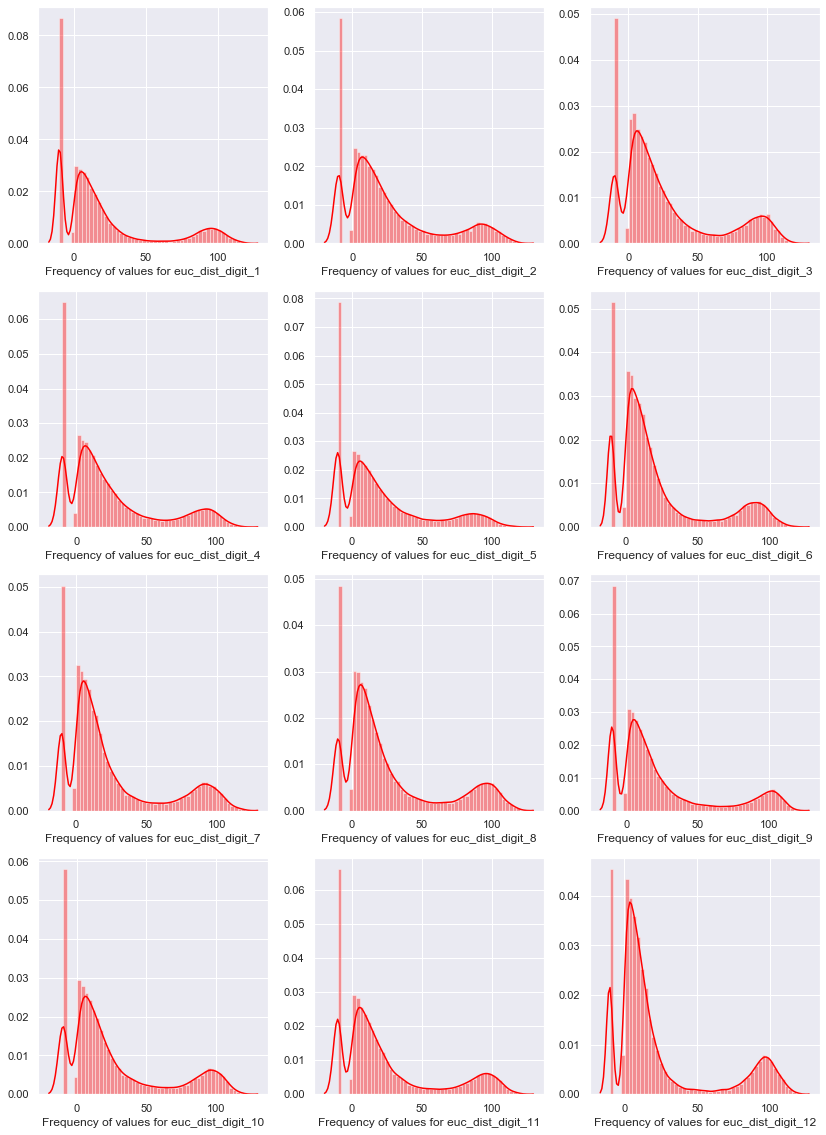In [ ]:
```#Let's look at Euclidean distance from center(512,512) to digits
#this feature can be calculated using Euclidean distance forumula for ideal and found digit positions with sqrt(a^2+b^2)

plt.figure()
fig, ax = plt.subplots(4, 3,figsize=(14, 20))
cont_features = [f"{i} dist from cen" for i in range(1,13)]
for i,feature in enumerate(cont_features):
plt.subplot(4, 3,i+1)
df[feature].fillna(-10,inplace=True)
sns.distplot(df[feature] , color='Red')
plt.xlabel(f"Frequency distribution of values for {feature}", fontsize=12);# plt.legend()
plt.show()
```
`<Figure size 432x288 with 0 Axes>`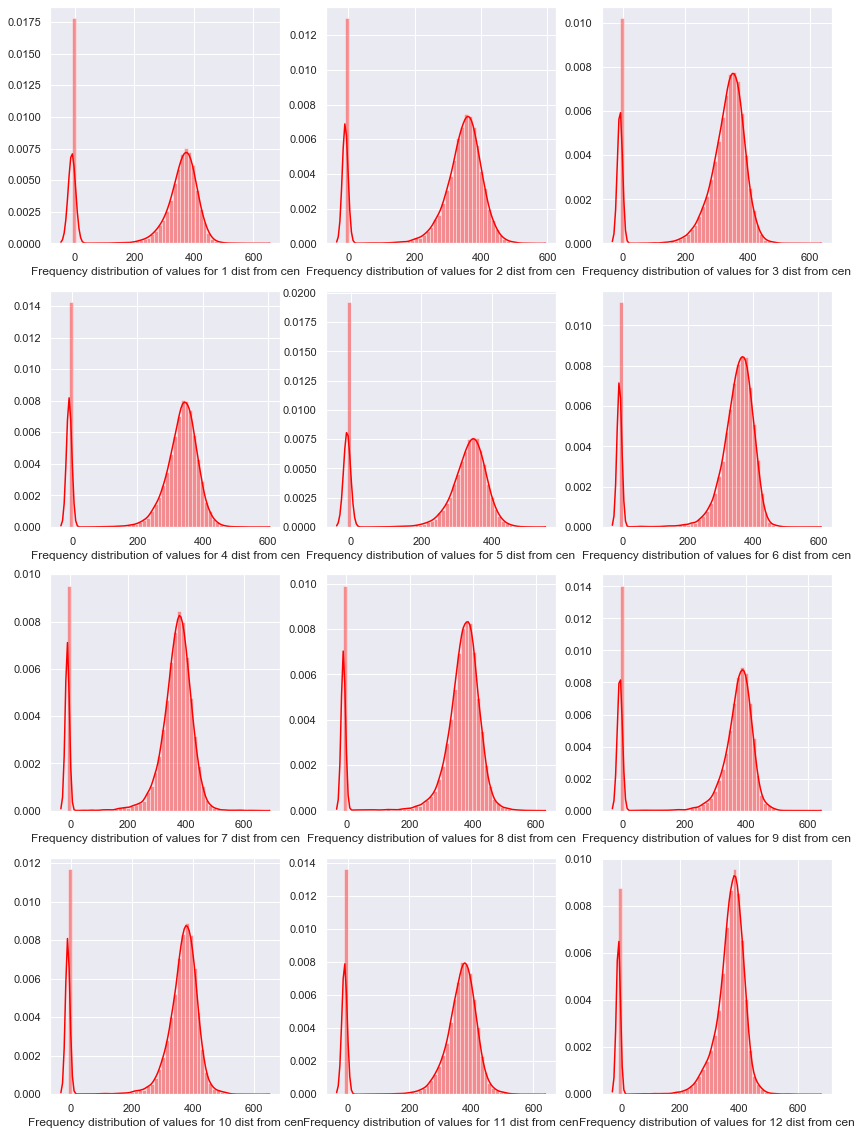The distribution seems to have variance around 200 with balanced gaussian distribution. Another thing to notice is that there are a lot of missing values in those variables.

In [ ]:
```#Next set of variables are area for each digit bounding boxes

plt.figure()
fig, ax = plt.subplots(4, 3,figsize=(14, 20))
cont_features = [f"area_digit_{i}" for i in range(1,13)]
for i,feature in enumerate(cont_features):
plt.subplot(4, 3,i+1)
df[feature].fillna(-1,inplace=True)
sns.distplot(df[feature] , color='Red')
plt.xlabel(f"Frequency distribution for {feature}", fontsize=12);# plt.legend()
plt.show()
```
`<Figure size 432x288 with 0 Axes>`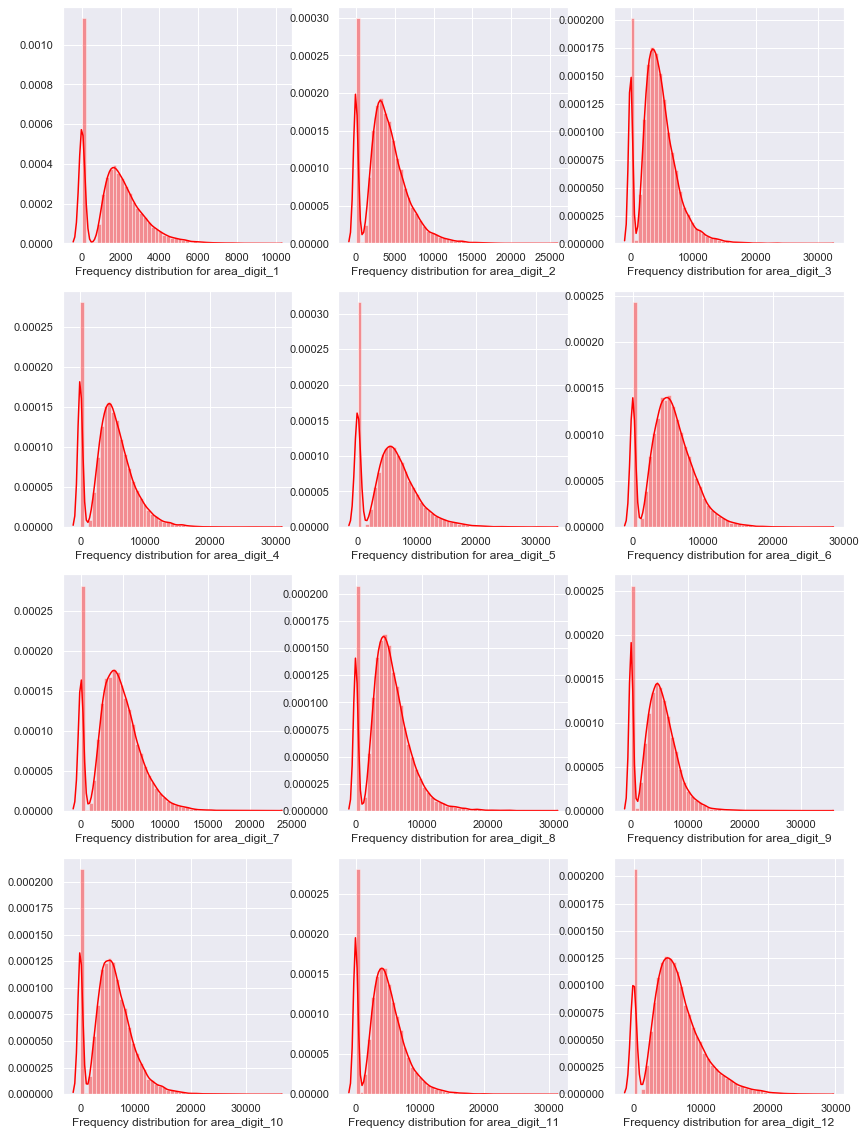We can notice the distributions have large variance and the distributions seem to be skewed. We may use some feature engineering to mkae it right.

In [ ]:
```#Next set of variables are height of each digit bounding boxes

plt.figure()
fig, ax = plt.subplots(4, 3,figsize=(14, 20))
cont_features = [f"height_digit_{i}" for i in range(1,13)]
for i,feature in enumerate(cont_features):
plt.subplot(4, 3,i+1)
df[feature].fillna(-1,inplace=True)
sns.distplot(df[feature] , color='Red')
plt.xlabel(f"Frequency distribution for {feature}", fontsize=12);# plt.legend()
plt.show()
```
`<Figure size 432x288 with 0 Axes>`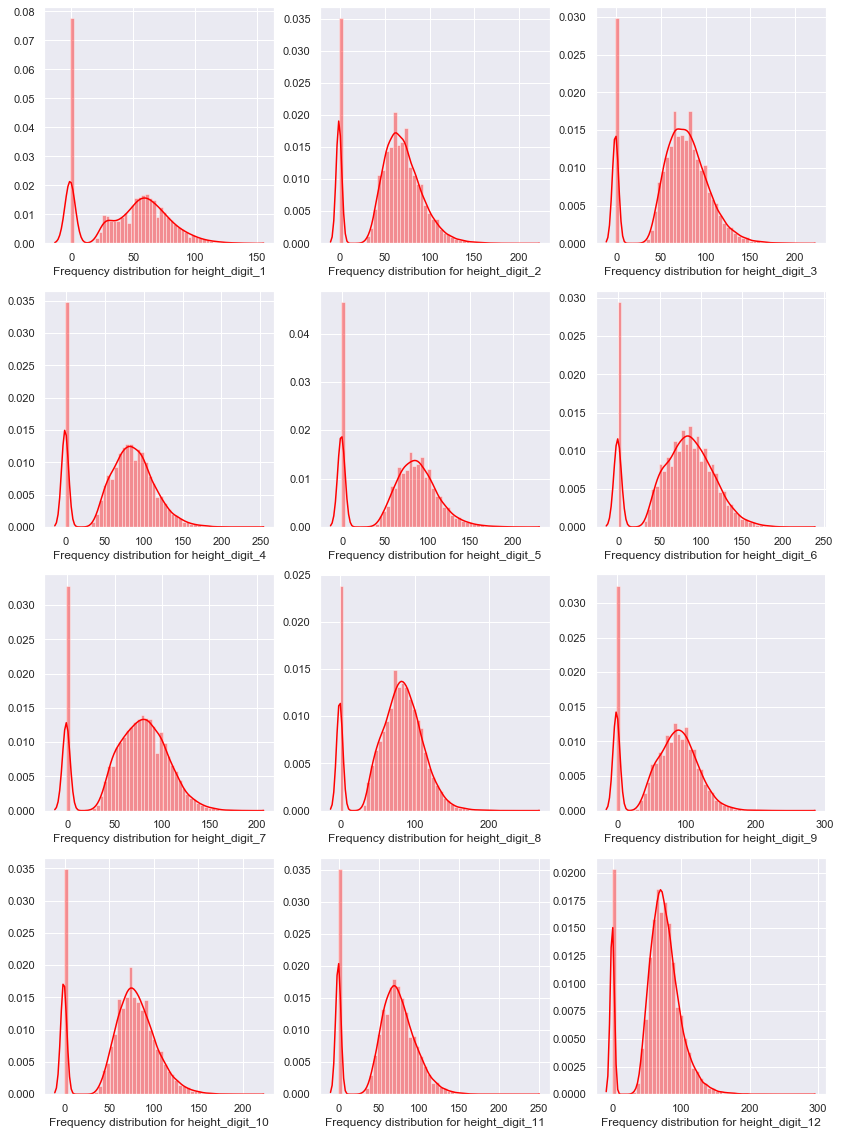There is a lot of variance in height of bounding boxes. This may explain the different sizes of bounding boxes as we can see the size will be diferent for some digits and 11, 12.

In [ ]:
```#Next set of variables are width for each digit bounding boxes

plt.figure()
fig, ax = plt.subplots(4, 3,figsize=(14, 20))
cont_features = [f"width_digit_{i}" for i in range(1,13)]
for i,feature in enumerate(cont_features):
plt.subplot(4, 3,i+1)
df[feature].fillna(-1,inplace=True)
sns.distplot(df[feature] , color='Red')
plt.xlabel(f"Frequency distribution for {feature}", fontsize=12); # plt.legend()
plt.show()
```
`<Figure size 432x288 with 0 Axes>`